## Overview

CimpleG, an R package to find (simple) CpG signatures.

## Installation

# Install directly from github:
devtools::install_github("costalab/CimpleG")

#  - ie navigating through your system
install.packages(file.choose(), repos = NULL, type = "source")
#  - ie given a path to a local source
# or
devtools::install_local("~/Downloads/CimpleG_0.0.5.XXXX.tar.gz")

## Getting started

library("CimpleG")

data(train_data)
data(train_targets)
data(test_data)
data(test_targets)

# check the train_targets table to see
# what other columns can be used as targets
# colnames(train_targets)

# mini example with just 4 target signatures
set.seed(42)
cimpleg_result <- CimpleG(
train_data = train_data,
train_targets = train_targets,
test_data = test_data,
test_targets = test_targets,
method = "CimpleG",
target_columns = c(
"neurons",
"glia",
"blood_cells",
"fibroblasts"
)
)

cimpleg_result$results # check generated signatures cimpleg_result$signatures
#>      neurons         glia  blood_cells  fibroblasts
#> "cg24548498" "cg14501977" "cg04785083" "cg03369247"

### Plot generated signatures


# adjust target names to match signature names

# check generated signatures
plt <- signature_plot(
cimpleg_result,
train_data,
train_targets,
sample_id_column = "gsm",
true_label_column = "cell_type"
)
print(plt$plot)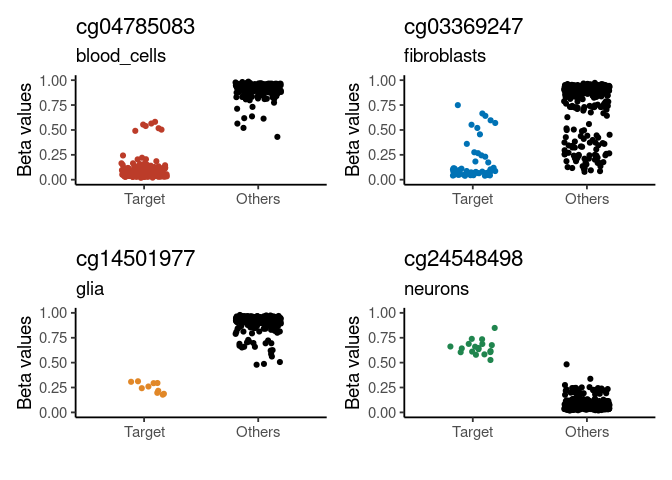## Difference of means vs Sum of variances (dmsv) plots ### basic plot plt <- diffmeans_sumvariance_plot( data = train_data, target_vector = train_targets$neurons == 1
)
print(plt)### adding color, highlighting selected features

df_dmeansvar <- compute_diffmeans_sumvar(
data = train_data,
target_vector = train_targets$neurons == 1 ) parab_param <- .7 df_dmeansvar$is_selected <- select_features(
x = df_dmeansvar$diff_means, y = df_dmeansvar$sum_variance,
a = parab_param
)

plt <- diffmeans_sumvariance_plot(
data = df_dmeansvar,
label_var1 = "Neurons",
color_all_points = "purple",
threshold_func = function(x, a) (a * x) ^ 2,
is_feature_selected_col = "is_selected",
func_factor = parab_param
)
print(plt)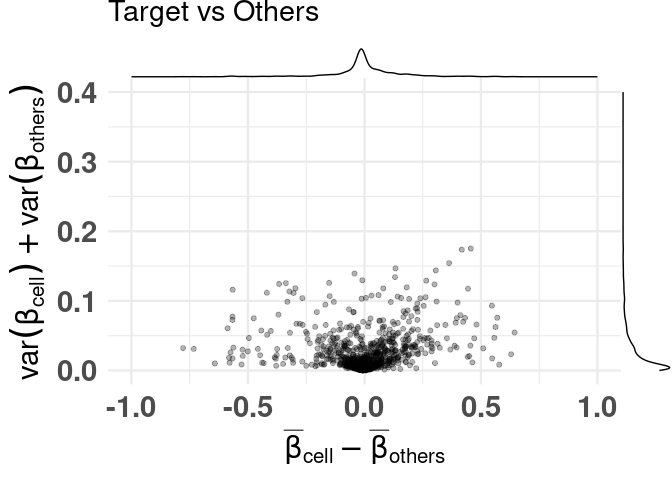### labeling specific features

plt <- diffmeans_sumvariance_plot(
data = df_dmeansvar,
feats_to_highlight = cimpleg_result$signatures ) print(plt)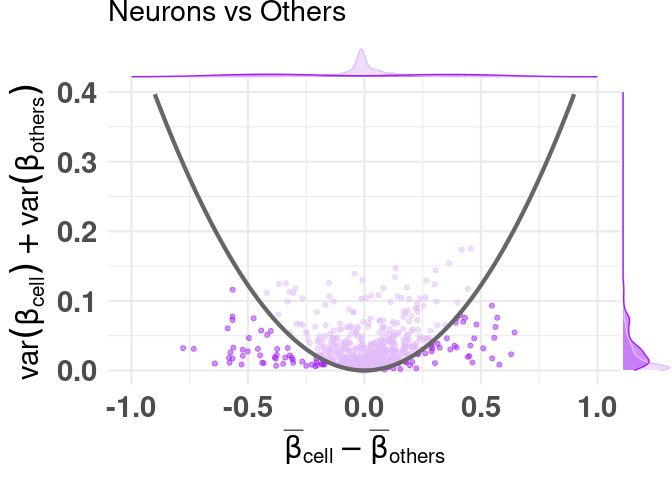## Deconvolution plots ### mini example with just 4 signatures deconv_result <- run_deconvolution( cpg_obj = cimpleg_result, new_data = test_data ) plt <- deconvolution_barplot( deconvoluted_data = deconv_result, meta_data = test_targets, sample_id = "gsm", true_label = "cell_type" ) print(plt$plot)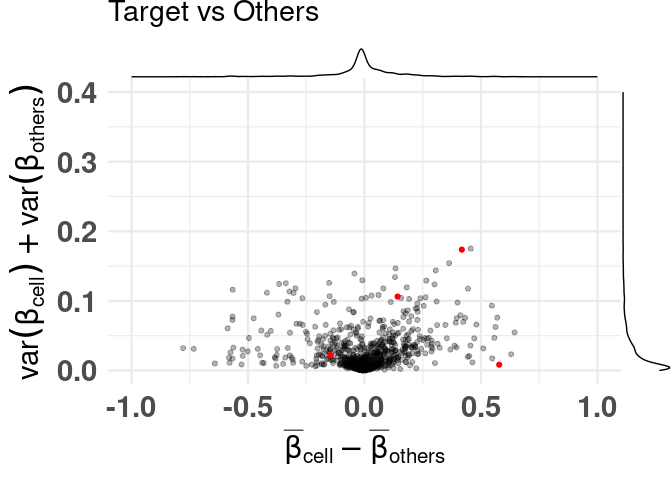### this example is a little more advanced

#### first lets create additional deconvolution results so that we can compare them

In this example, we’ll create two additional models made with CimpleG. One using only hypermethylated signatures, and the other using 3 CpGs per signature instead of just one.


set.seed(42)
cimpleg_hyper <- CimpleG(
train_data = train_data,
train_targets = train_targets,
test_data = test_data,
test_targets = test_targets,
method = "CimpleG",
pred_type = "hyper",
target_columns = c(
"neurons",
"glia",
"blood_cells",
"fibroblasts"
)
)
#> Training for target 'neurons' with 'CimpleG' has finished.: 0.652 sec elapsed
#> Training for target 'glia' with 'CimpleG' has finished.: 0.378 sec elapsed
#> Training for target 'blood_cells' with 'CimpleG' has finished.: 0.422 sec elapsed
#> Training for target 'fibroblasts' with 'CimpleG' has finished.: 0.371 sec elapsed

deconv_hyper <- run_deconvolution(
cpg_obj = cimpleg_hyper,
new_data = test_data
)

set.seed(42)
cimpleg_3sigs <- CimpleG(
train_data = train_data,
train_targets = train_targets,
test_data = test_data,
test_targets = test_targets,
method = "CimpleG",
n_sigs = 3,
target_columns = c(
"neurons",
"glia",
"blood_cells",
"fibroblasts"
)
)
#> Training for target 'neurons' with 'CimpleG' has finished.: 0.499 sec elapsed
#> Training for target 'glia' with 'CimpleG' has finished.: 0.72 sec elapsed
#> Training for target 'blood_cells' with 'CimpleG' has finished.: 0.482 sec elapsed
#> Training for target 'fibroblasts' with 'CimpleG' has finished.: 0.513 sec elapsed

deconv_3sigs <- run_deconvolution(
cpg_obj = cimpleg_3sigs,
new_data = test_data
)

#### remember this is just an example, the results themselves are meaningless!

deconv_3sigs$prop_3sigs <- deconv_3sigs$proportion
deconv_hyper$prop_hyper <- deconv_hyper$proportion
deconv_result$prop_cimpleg <- deconv_result$proportion

dummy_deconvolution_data <-
deconv_result |>
dplyr::mutate(true_vals = proportion + runif(nrow(deconv_result), min=-0.1,max=0.1)) |>
dplyr::select(cell_type,sample_id,prop_cimpleg,true_vals) |>
dplyr::left_join(deconv_hyper |> dplyr::select(-proportion), by=c("sample_id","cell_type")) |>
dplyr::left_join(deconv_3sigs |> dplyr::select(-proportion), by=c("sample_id","cell_type")) |>
dplyr::mutate_if(is.numeric, function(x){ifelse(x<0,0,x)}) |>
dplyr::mutate_if(is.numeric, function(x){ifelse(x>1,1,x)}) |>
tibble::as_tibble()

#### first we can check how the true values compare against the predicted values

scatter_plts <- CimpleG:::deconv_pred_obs_plot(
deconv_df = dummy_deconvolution_data,
true_values_col = "true_vals",
predicted_cols = c("prop_cimpleg","prop_hyper","prop_3sigs"),
sample_id_col = "sample_id",
group_col= "cell_type"
)
scatter_panel <- scatter_plts |> patchwork::wrap_plots(ncol=1)

print(scatter_panel)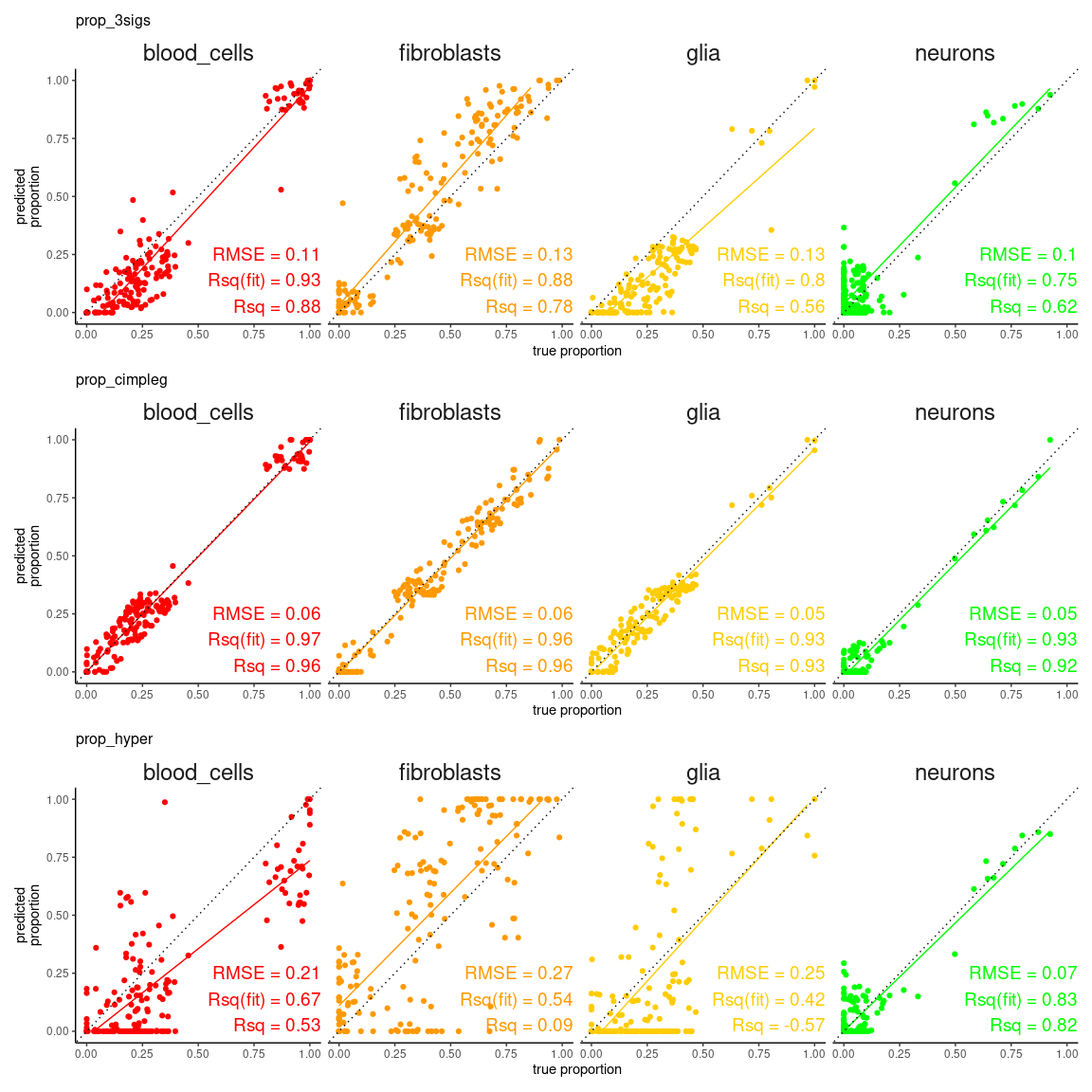#### now, more interestingly, we can see in detail and rank one of the measures used to evaluate the deconvolution results

rank_plts <- CimpleG:::deconv_ranking_plot(
deconv_df = dummy_deconvolution_data,
true_values_col = "true_vals",
predicted_cols = c("prop_cimpleg","prop_hyper","prop_3sigs"),
sample_id_col = "sample_id",
group_col= "cell_type",
metrics = "rmse"
)
rank_panel <- list(rank_plts$perf_boxplt[],rank_plts$nemenyi_plt[]) |> patchwork::wrap_plots()

print(rank_panel)